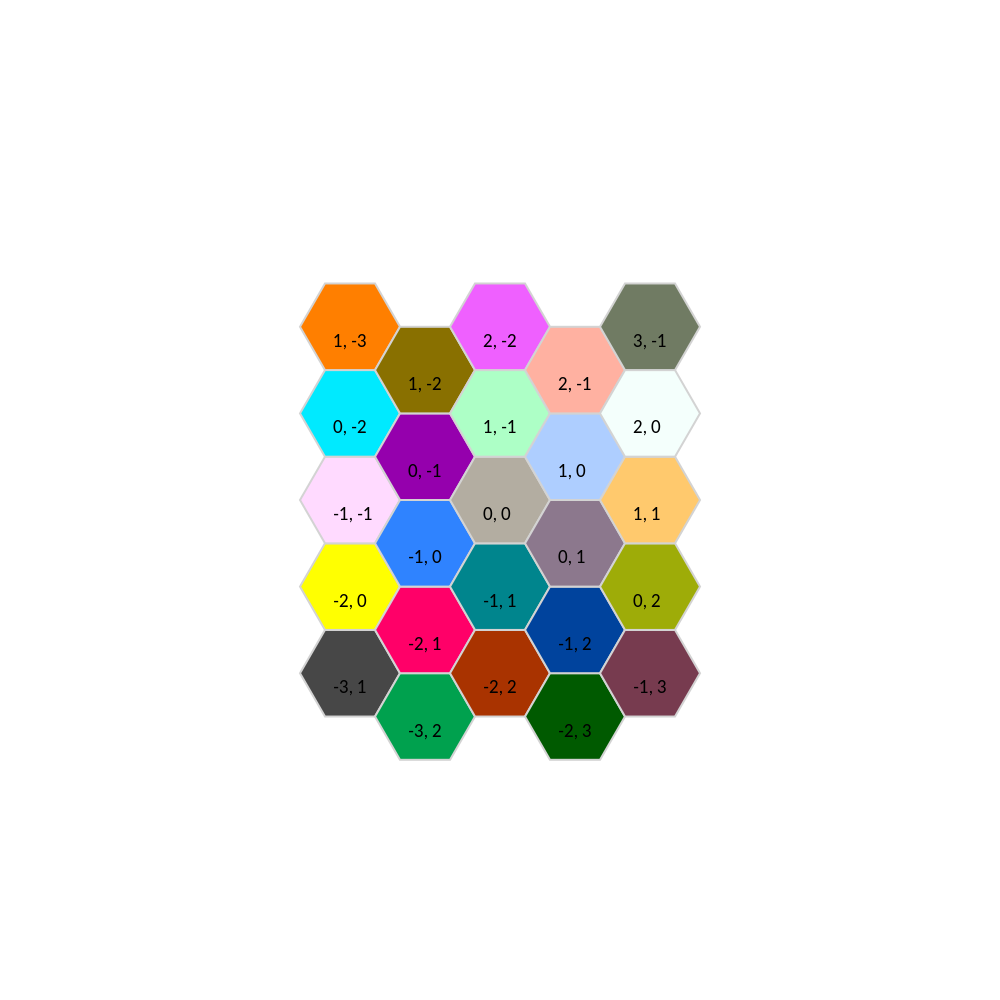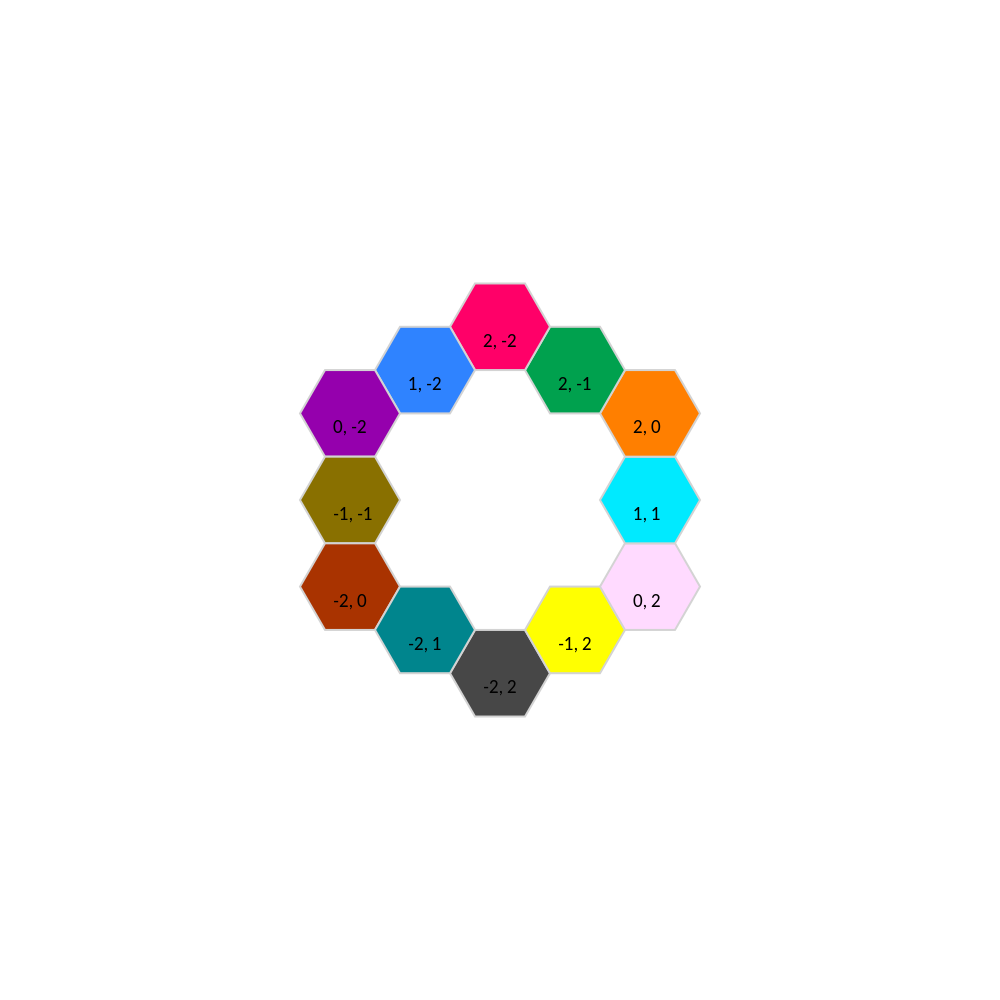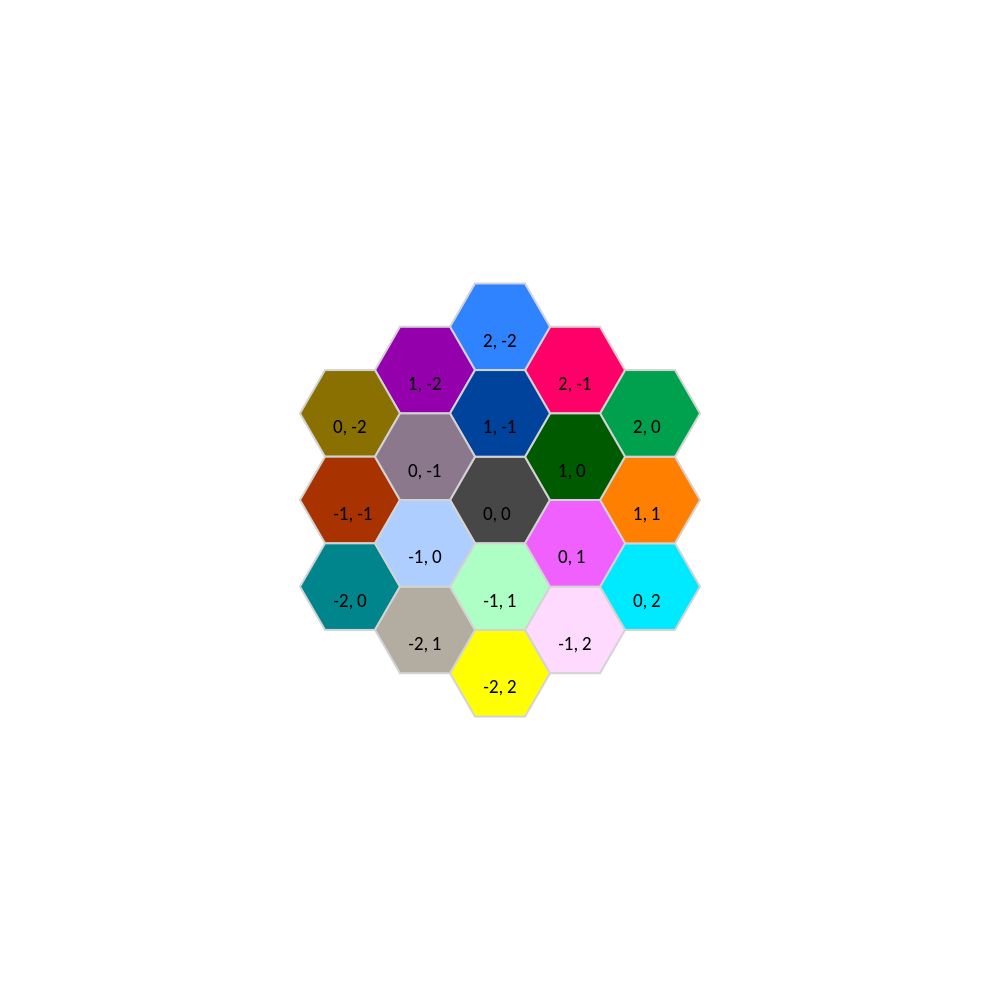# Repeating Structures

The Repeat module contains functions for creating regular repeated patterns. This could be pixels in a display grid, or mirrors in an active optics telescope. Repeated patterns are defined by creating an object that inherits from the abstract type Basis.

Subtypes supporting the Basis interface should implement these functions:

Returns the neighbors in ring n surrounding centerpoint, excluding centerpoint

neighbors(::Type{B},centerpoint::Tuple{T,T},neighborhoodsize::Int) where{T<:Real,B<:Basis}

Returns the lattice basis vectors that define the lattice

basis(a::S) where{S<:Basis}

Returns the vertices of the unit polygon for the basis that tiles the plane

tilevertices(a::S) where{S<:Basis}

A lattice is described by a set of lattice vectors eᵢ which are stored in a Basis object. You can create bases in any dimension. Points in the lattice are indexed by integer coordinates. These lattice coordinates can be converted to Cartesian coordinates by indexing the LatticeBasis object.

using OpticSim, OpticSim.Repeat
a = LatticeBasis((1.0,5.0),(0.0,1.0))
a[3,3]
2-element StaticArrays.SVector{2, Float64} with indices SOneTo(2):
3.0
18.0

The Lattice points are defined by a weighted sum of the basis vectors:

latticepoint = ∑αᵢ*eᵢ

where the αᵢ are integer weights.

The HexBasis1 constructor defines a symmetric basis for hexagonal lattices

using OpticSim, OpticSim.Repeat
basis(HexBasis1())

The rectangularlattice function creates a rectangular lattice basis.

There are a few visualization functions for special 2D lattices. Vis.drawcells draws a set of hexagonal cells. Using Repeat.hexcellsinbox we can draw all the hexagonal cells that fit in a rectangular box:

using OpticSim
Vis.drawcells(Repeat.HexBasis1(),50,Repeat.hexcellsinbox(2,2))There is also a function to compute the n rings of a cell x, i.e., the cells which can be reached by taking no more than n steps along the lattice from x:

using OpticSim
Vis.drawcells(Repeat.HexBasis1(),50,Repeat.neighbors(Repeat.HexBasis1,(0,0),2))You can also draw all the cells contained within an n ring:

using OpticSim
Vis.drawcells(Repeat.HexBasis1(),50,Repeat.region(Repeat.HexBasis1,(0,0),2))